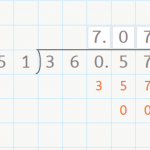Smartick is a fun way to learn math!# I want to learn about: Decimals

Learn about decimals with Smartick. Exercises, tutorials problems, and teaching resources about decimals for elementary-age children. Here you will find posts about decimals to make learning mathematics easier and more fun.

Jun10

## Dividing a Decimal by a Whole NumberDividing a Decimal by a Whole Number Hello everyone! In this week’s post, we are going to talk about dividing a decimal by a whole number. You already know how to divide a whole number by a whole number by now, right? If not, check out our posts about dividing by a one-digit number here, […]